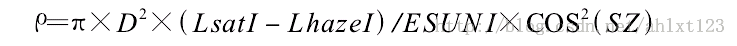# python中使用opencv库来进行简单的气象学遥感影像计算

opencv的全称是open source computer vision library，是一个跨平台的计算机视觉库。opencv是由英特尔公司发起并参与开发，以bsd许可证授权发行，可以在商业和研究领域中免费使用。opencv可用于开发实时的图像处理、计算机视觉以及模式识别程序。该程序库也可以使用英特尔公司的ipp进行加速处理。
opencv用c++语言编写，它的主要接口也是c++语言，但是依然保留了大量的c语言接口。该库也有大量的python, java and matlab/octave (版本2.5)的接口。这些语言的api接口函数可以通过在线文档获得。现在也提供对于c#, ch,ruby的支持。

1、遥感影像的光谱辐射定标def computl(gain,dn,bias):
return (gain*dn+bias)

2、遥感影像的大气校正#esun
esuni71=196.9
#
lmini=-6.2
lmax=293.7
#
qcal=1
qmax=255
limin=lmini+(qcal*(lmax-lmini)/qmax)
li=(0.01*esuni71*cos*cos)/(math.pi*d*d)
lhazel=limin-li

3、计算遥感影像的反射率（jd为遥感成像的儒略日(julian day)，计算公式为：

jd=k-32075+1461*(i+4800+(j-14)/12)/4+367*(j-2-(j-14)/12*12)/12-3*((i+4900+(j-14)/12)/100)/4

i、j、k分别为年、月、日

import cv2
import numpy as np
import math
#图像格式转换
img10=cv2.cvtcolor(img1,cv2.color_bgr2gray)
#计算jd
i=2003
j=2
k=20
jd=k-32075+1461*(i+4800+ (j-14)/12)/4+367*(j-2-(j-14)/12*12)/12-3*((i+4900+(j-14)/12)/100)/4
#设置esuni值
esuni71=196.9
#计算日地距离d
d=1-0.01674*math.cos((0.9856*(jd-4)*math.pi/180))
#计算太阳天顶角
inter=(math.pi*d*d)/(esuni71*cos*cos)
#大气校正参数设置
lmini=-6.2
lmax=293.7
qcal=1
qmax=255
limin=lmini+(qcal*(lmax-lmini)/qmax)
li=(0.01*esuni71*cos*cos)/(math.pi*d*d)
lhazel=limin-li
def copy(img,new1):
new1= np.zeros(img.shape,dtype=’uint16′)
new1[:,:] = img[:,:]
def computl(gain,dn,bias):
return (gain*dn+bias)
if __name__ == ‘__main__’:
print ‘d=’,d
print ‘coszs=’,cos
print ‘lhazel=’,lhazel
#计算图像反射率
result=np.zeros(img.shape,dtype=’uint16′)
for i in range(0,img.shape(1)):
for j in range(0,img.shape(0)):
lsat=computl(1.18070871,img10[i,j],-7.38070852)
result[i,j]=inter*(lsat-lhazel)*1000
#保存图像
cv2.imwrite(“f:\\result.tif”, result)
cv2.namedwindow(“image”)
cv2.imshow(“image”, result)
cv2.waitkey(0)

Posted in 未分类